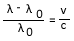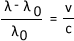## Velocity of a Galaxy

16.3 - Be able to use the formula:where λ is the observed wavelength, λ0 is the emitted wavelength, v is the radial velocity of the source, c is the speed of light

This equation measure the radial velocity of a galaxy - how fast it is moving.

The symbol you are currently puzzled by (unless you are very clever) is the greek letter 'lambda' - λ- which symbolises 'wavelength'.

Measuring radiation from a galaxy gives us its wavelength.

That was the easy bit. Let's split this up ...

λ = observed galaxy wavelength
λ0 = rest wavelength of the galaxy
(see below)

v = the velocity of a galaxy
c = the speed of light (300,000 km/s
)

The rest wavelength is observed when there is no relative motion between the galaxy and the observer.

The value of these wavelengths is measured in Angstroms (Å).

ExampleAn absorbtion line of a galaxy is measured at 4040Å and 4000Å at rest

λ - λ0 is 4040 - 4000 = 40

Divide 40 by λ0 (4000) is 0.01

Velocity = 0.01

0.01 x c is 0.01 x 300,000 = 3000

Galaxy moving at a radial velocity of 3000 km/s